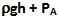## Sunday, June 28, 2009

### Irodov Problem 1.329We assume that just before the tube in the tank the water is still i.e its velocity is 0. Let the velocity of water as it exits out of the spout be vs. The internal pressure of water just before the tube in the tank is given by the pressure due to water column and the atmospheric pressure PA as.
As the water exists out of the spout the pressure is equal to the atmospheric pressure. Applying Bernoulli's equation for just before the tube and as it exists out of the tube we can write,Now let us consider a cross-section within the tube that has cross-section area a. Let the velocity of the water at this point be v. Since the rate of volume of the water flowing out the tube must be equal at all cross-sections within the tube we have,Let the internal pressure at this cross-section within the tube be P. Appllying Bernoulli's equation at this cross-section within the tube and at the exit point of the tube we have,The water exerts pressure P normal to the surface of the tube outwards and the atmosphere exerts pressure PA pushing it inwards. The net pressure exerted on the tube outwards is thus P-PA. The horizontal component of the force due to this pressure (dF) that is trying to rip this section of the tube out of the tank is given by the pressure times the vertical component of the area of the tube given by,Unknown said...

In (Q)1.329 I have a doubt. beside atmospheric pressure I think there is one more pressure responsible for pushing the nozzle inward and that pressure is the pressure exerted by outcoming water from the nozzle,as we have seen in 1.328.But in this solution this pressure is not taken into account. I want to know am i right?

Krishna Kant Chintalapudi said...

This is a very good question.

First let us understand as to why this reaction force due to water jetting out arises in problems 1.326-1.328. The back pressure exerted by water coming out of the nozzle in problems, is similar that of jet propulsion. The reason this reaction arises is because F = d(mv)dt = (dm/dt)v. As the water flows out, the total mass of water in the tank changes as a rate dm/dt = density.A.v. A is the cross-section area of the nozzle and v is the velocity of the water. So Pressure = F/A = (density)v.v.

In Problem 1.329, the question is to find out the force that is trying to rip the nozzle out, so we have to consider forces acting on the nozzle only and not on the entire (tank+zozzle) system. If you consider just the nozzle - at any given point of time the total mass of water within the nozzle is always constant i.e. dm/dt = 0 for the nozzle. Thus, this means that if you consider the forces acting just on the nozzle, there is no reaction force due to jetting of the water!!

Basically water is simply passing through and through the nozzle (entering at one end and leaving at the other) similar to water passing through some pipe. There will not be no jetting reaction on the pipe in such cases. You can also think of this as water entering the pipe generates a force that is exactly canceled by the force generated by exiting water.

Hope this explanation helps.

Unknown said...

Thank you. my doubt is clear now.But in future if I'm having any doubt,I will look here for help.